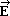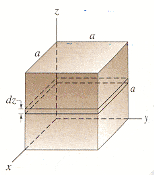# Calculate net charge with nonuniform electric field

## Homework Statement

In a cubical volume, 1.05 m on a side, the electric field is given by the formula below, where E0 = 1.25 N/C and a = 1.05 m.= E0(1 + z/a) i + E0(z/a) j

The cube has its sides parallel to the coordinate axes, see the figure. Determine the net charge within the cube.## Homework Equations

φe = ∫E⋅dA = qenc0

## The Attempt at a Solution

[/B]
So I know that I need to calculate the net flux through all the 6 faces of the cube in order to solve for qenc. I know that φ+z and φ-z are equal to 0.

I think I am doing something wrong because it seems like they would cancel out?

φ+x = E0 a2 ∫ (1+z/a) dz
φ-x = -E0 a2 ∫ (1+z/a) dz
φ+y = E0 a2 ∫ (z/a) dz
φ-y = -E0 a2 ∫ (z/a) dz

Also, I would evaluate the integrals from 0 to a right?

Last edited:

SammyS
Staff Emeritus
Homework Helper
Gold Member

## Homework Statement

In a cubical volume, 1.05 m on a side, the electric field is given by the formula below, where E0 = 1.25 N/C and a = 1.05 m.= E0(1 + z/a) i + E0(z/a) j

The cube has its sides parallel to the coordinate axes, see the figure. Determine the net charge within the cube.## Homework Equations

φe = ∫E⋅dA = qenc0

## The Attempt at a Solution

[/B]
So I know that I need to calculate the net flux through all the 6 faces of the cube in order to solve for qenc. I know that φ+z and φ-z are equal to 0.

I think I am doing something wrong because it seems like they would cancel out?

φ+x = E0 a2 ∫ (1+z/a) dz
φ-x = -E0 a2 ∫ (1+z/a) dz
φ+y = E0 a2 ∫ (z/a) dz
φ-y = -E0 a2 ∫ (z/a) dz

Also, I would evaluate the integrals from 0 to a right?
You have done a lot correct.

The units will be wrong.

Where does a2 come from ?

Hello!

I would evaluate the integrals from 0 to a right?
Yes.
I think I am doing something wrong because it seems like they would cancel out?
You don't think this is possible? Notice that for a particular ##z##, ##E## remains constant throughout the object. Also, what is the definition of flux?

You have done a lot correct.

The units will be wrong.

Where does a2 come from ?

I think I was getting confused between ∫ E⋅dA and E⋅A, but since I'm doing the integral one then I don't need a2. So,

φ+x = E0 ∫ (1+z/a) dz
φ-x = -E0 ∫ (1+z/a) dz
φ+y = E0 ∫ (z/a) dz
φ-y = -E0 ∫ (z/a) dz

from 0 to a.

SammyS
Staff Emeritus
Homework Helper
Gold Member
I think I was getting confused between ∫ E⋅dA and E⋅A, but since I'm doing the integral one then I don't need a2. So,

φ+x = E0 ∫ (1+z/a) dz
φ-x = -E0 ∫ (1+z/a) dz
φ+y = E0 ∫ (z/a) dz
φ-y = -E0 ∫ (z/a) dz

from 0 to a.
The units are also incorrect this time.

You need to integrate over y for some & over x for others. Since E is independent of x & y the result of those is easy.